# IIT JAM Chemistry - MCQ Test 2

## 60 Questions MCQ Test MOCK Test Series for IIT JAM Chemistry | IIT JAM Chemistry - MCQ Test 2

Description
This mock test of IIT JAM Chemistry - MCQ Test 2 for Chemistry helps you for every Chemistry entrance exam. This contains 60 Multiple Choice Questions for Chemistry IIT JAM Chemistry - MCQ Test 2 (mcq) to study with solutions a complete question bank. The solved questions answers in this IIT JAM Chemistry - MCQ Test 2 quiz give you a good mix of easy questions and tough questions. Chemistry students definitely take this IIT JAM Chemistry - MCQ Test 2 exercise for a better result in the exam. You can find other IIT JAM Chemistry - MCQ Test 2 extra questions, long questions & short questions for Chemistry on EduRev as well by searching above.
*Multiple options can be correct
QUESTION: 1

### The order of decreasing energy among covalent bond (CB), dipole-dipole interaction (DDI) and London dispersion forces (LDF) is:

Solution:
*Multiple options can be correct
QUESTION: 2

### T50 (half-life period) of first order reaction is 10 min. Starting with 10 mol L-1, rate after 20 min is:

Solution:
*Multiple options can be correct
QUESTION: 3

### The set representing the correct order of ionic radius is:

Solution:
*Multiple options can be correct
QUESTION: 4

Which of the following magnetic moment values will correspond to highest ionization energy form Mn species:

Solution:
*Multiple options can be correct
QUESTION: 5

For the reaction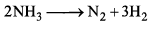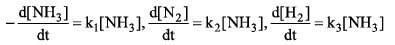Then relation between k1, k2 and k3 is

Solution:
*Multiple options can be correct
QUESTION: 6

Efficiency of Carnot’s engine is 100 % when

Solution:
*Multiple options can be correct
QUESTION: 7

Arrange the following compounds in order of Ca—Cb bond length: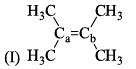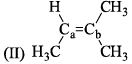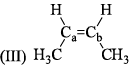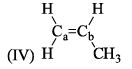Solution:
*Multiple options can be correct
QUESTION: 8

All the following are the resonance structure of one another except:

Solution:
*Multiple options can be correct
QUESTION: 9

Bombardment of aluminium by α-particle leads to its artificial disintegration in two ways (i) and (ii) as shown. Product X, Y and Z respectively are: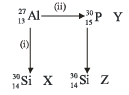Solution:
*Multiple options can be correct
QUESTION: 10

Heat of dissociation of CH3COOH is 0.005 kcal g-1, hence enthalpy change when 1 mole of Ca(OH)is completely neutralized by CH3COOH is:

Solution:
*Multiple options can be correct
QUESTION: 11

The heat released on neutralization of CsOH with all strong acids is 13.4 kcal mol-1. The heat released on neutralization of CsOH with HF (weak acid) is 16.4 kcal mol-1. ΔH° of ionization of HF in water is:

Solution:
*Multiple options can be correct
QUESTION: 12

At low pressure, that Vander Waal’s equation is reduced to:

Solution:
*Multiple options can be correct
QUESTION: 13

Correct order of stability of the following carbocation is: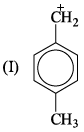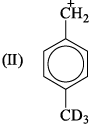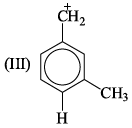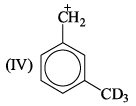Solution:

At metaposition, hyperconjugation do not take place.

Inductive effect order −H<−D i.e R−CH3​<R−CD3 (Stability)

Hyperconjugation order −H<−D i.e R−CH3>R−CD3​ (Stability)

and Hyperconjugated compound is always more stable than inductive effect.

Therefore, Order is I>II>IV>III

*Multiple options can be correct
QUESTION: 14

The decay modes of 14C and 14O are:

Solution:
*Multiple options can be correct
QUESTION: 15

If 3/4 quantity of a radioactive element disintegrates in two hours its half- life would be:

Solution:
*Multiple options can be correct
QUESTION: 16

What is the stereochemical relationship between the following compound: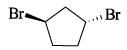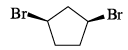Solution:
*Multiple options can be correct
QUESTION: 17

Among the structures given below, the most stable conformation for the following compound is: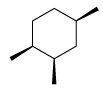Solution:
*Multiple options can be correct
QUESTION: 18

Following configuration of the tartaric acid represents: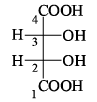Solution:
*Multiple options can be correct
QUESTION: 19

The gauche conformation (θ = 60°) of n-butane possess:

Solution:
*Multiple options can be correct
QUESTION: 20

Rate of formation of SO3 in the following reaction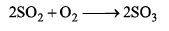is 100g min-1. Hence, rate of disappearance of O2 is:

Solution:

Rate of a reaction is defined as the rate of change of concentration per unit time.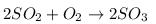The rate in terms of reactants is given as negative as the concentration of reactants is decreasing with time whereas the rate in terms of products is given as positive as the concentration of products is increasing with time.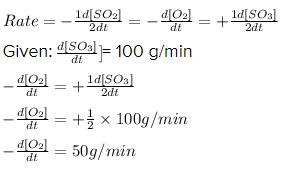*Multiple options can be correct
QUESTION: 21

Any gas shows maximum deviation from ideal gas at:

Solution:
*Multiple options can be correct
QUESTION: 22

The de-Broglie wavelength of the electron in the ground state of hydrogen atom is ? Given: KE = 13.6 eV, 1 eV = 1.6*10 -19J.

Solution:
*Multiple options can be correct
QUESTION: 23

For a first order homogenous gaseous reaction,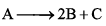then initial pressure was P, while total pressure after time ‘t’ was Pt. The write expression for the rate constants k in terms of Pi, Pt and t is:

Solution:
*Multiple options can be correct
QUESTION: 24

In which of the following reactions, hydrogen peroxide acts as on oxidizing agent:

Solution:
*Multiple options can be correct
QUESTION: 25

Heat of neutralization of H2C2O4 (oxalic acid) is - 2 6 kcal mol-1. Hence dissociation energy of: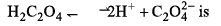Solution:
*Multiple options can be correct
QUESTION: 26

Which is the correct order of dipole moments of the compounds: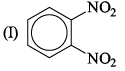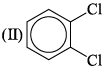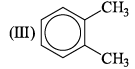Solution:
*Multiple options can be correct
QUESTION: 27

Which carbocation is the most stabilized:

Solution:
*Multiple options can be correct
QUESTION: 28

Nodal plane in the π bond is located in:

Solution:
*Multiple options can be correct
QUESTION: 29

The correct order of increasing bond angles in the following triatomic species

Solution:
*Multiple options can be correct
QUESTION: 30

The schrodinger wave equation for hydrogen atom is: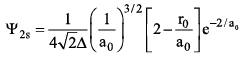Solution:
*Multiple options can be correct
QUESTION: 31

For the reaction: H3PO3 + Ba(OH)→ BaHPO3 + 2H2O The correct statement(s) is/are

Solution:
*Multiple options can be correct
QUESTION: 32

Select incorrect statement(s):

Solution:

(b) Compressibility factor of an ideal gas is 1.
(c) Real gas behaves like ideal gas at high temperature and low pressure.

*Multiple options can be correct
QUESTION: 33

Which of the following set(s) of quantum numbers is(are) not allowed:

Solution:
*Multiple options can be correct
QUESTION: 34

A radioactive element X emits α-particles followed by 2 p-particles in the successive steps as: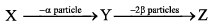Which of the following statement(s) is/are correct?

Solution:
*Multiple options can be correct
QUESTION: 35

Identify which of the structures below are meso structures:

Solution:
*Multiple options can be correct
QUESTION: 36

Select the correct statements, out of I, II and III for zeroth order reaction.
(I) Quantity of the product formed, is directly proportional to time.
(II) Larger the initial concentration of the reactant greater the half-life period
(III) If 50% reaction takes place in 100 min, 75% reaction take place in 150 min.

Solution:
*Multiple options can be correct
QUESTION: 37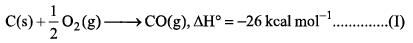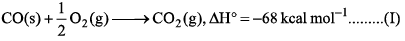Which is/are correct statements):

Solution:
*Multiple options can be correct
QUESTION: 38

The enthalpy of neutralization of which of the following acids and bases is nearly -13.6 kcal:

Solution:
*Multiple options can be correct
QUESTION: 39

The following is (are) endothermic reaction:

Solution:
*Multiple options can be correct
QUESTION: 40

Hydrogen bonding plays a central role in the following phenomena:

Solution: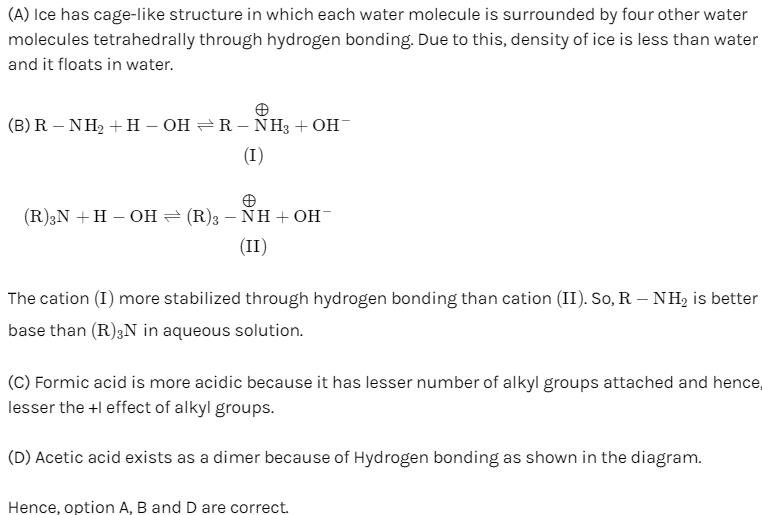*Answer can only contain numeric values
QUESTION: 41

What is the strength (in g/litre) of a solution of H2SO4, 12 mL of which neutralized 15 mL of N/10 NaOH solution:

Solution:
*Answer can only contain numeric values
QUESTION: 42

In an ideal monoatomic gas, the speed of sound is given by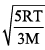. If the speed of sound in argon at 25°C us 1245 km h-1, the root mean square velocity (in ms-1) is...........?

Solution:
*Answer can only contain numeric values
QUESTION: 43

Volume (mL) of H2C2O4. 2H2O solution to prepare 0.10 M from 1.575g of it is ?

Solution:
*Answer can only contain numeric values
QUESTION: 44

The effective nuclear charge of helium atom is 1.7. The first ionization energy of helium atom (in eV) is ....... ?

Solution:
*Answer can only contain numeric values
QUESTION: 45

The standard molar enthalpy of formation of molecular oxygen at 298 K is zero. Thus its value  (in KJ/mol) at 315 K is...... ? Given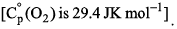Solution:
*Answer can only contain numeric values
QUESTION: 46

The amount of heat (in kJ) evolved when 500 cm3 of 0.1 M HCl is mixed with 200 cm3 of 0.2M NaOH is.......?

Solution:
*Answer can only contain numeric values
QUESTION: 47

Find out the double bond equivalent (DBE) value of the given following compound: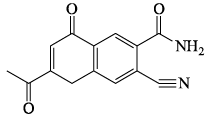Solution:
*Answer can only contain numeric values
QUESTION: 48

A mixture of 50.0 mL o f NH3 and 60.0 mL of Ogas reacts as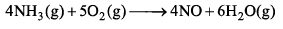If all the gases are at the same temperature and pressure and the reaction continuous until one of the gases is completely consumed, what volume (in mL) of water vapour is produced.

Solution:
*Answer can only contain numeric values
QUESTION: 49

How many of the following inter halogen species have 2 lone pairs of electrons on the central atom? ClF3, ClF2-, CIF5 ICl2- and XeF2

Solution:

Chlorine trifluoride has 5 regions of electron density around the central chlorine atom (3 bonds and 2 lone pairs). These are arranged in a trigonal bipyramidal shape with a 175° F(axial)-Cl-F(axial) bond angle.
In a ClF5 molecule, chlorine is the central atom featuring five single bonds with fluorine atoms. Since chlorine has 7 electrons in the valence shell, the remaining two electrons form a lone pair.

*Answer can only contain numeric values
QUESTION: 50

For the radioactive isotope 131I, the time required for 50% disintegration is 8 days. The time required for the 99.9% disintegration of 5.5g of 131I is __________ days, (up to one decimal place):

Solution:
*Answer can only contain numeric values
QUESTION: 51

The number of resonance structures for the compound is: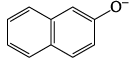Solution:
*Answer can only contain numeric values
QUESTION: 52

The standard enthalpy of formation of NH3 is -4 6 .0 kJ mol -1. If the enthalpy of formation of H2 from its atoms is —436 kJ mol -1 and that of N2 is -712 kJ mol the average bond enthalpy of N—H bond in NH3 is: (in kJ/mol)

Solution:
*Answer can only contain numeric values
QUESTION: 53

The ΔH° for the reaction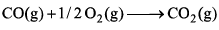at 400 K in kJ mol-1 is ___________________.

Given at 298 K: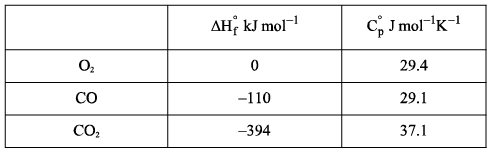Solution: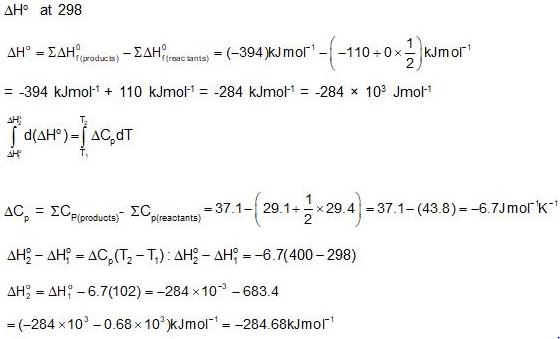*Answer can only contain numeric values
QUESTION: 54

One mole of Al4C3(s) reacts with H2O in a closed vessel at 27°C against atmospheric pressure. Work done (in cal) is....?

Solution:
*Answer can only contain numeric values
QUESTION: 55

The total number of compounds which show -M effects: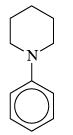;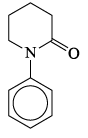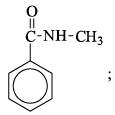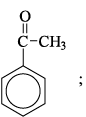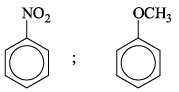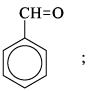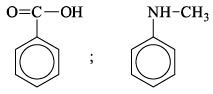Solution:
*Answer can only contain numeric values
QUESTION: 56

What will be the longest wavelength line in Balmer’s series of spectrum: (in nm)

Solution:

The longest wavelength means the lowest energy. We know that relation for wavelength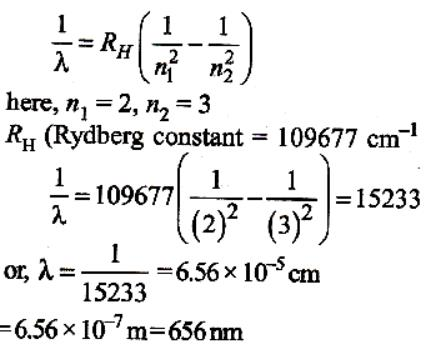*Answer can only contain numeric values
QUESTION: 57

N2 + 3H2 —> 2NH3. 1 mole N2 and 4 moles H2 are taken in 15 L flask at 27°C. After complete conversion of N2 into NH3, 5L of H2O is added. Pressure set up in the flask is: (in atm)

Solution:
*Answer can only contain numeric values
QUESTION: 58

The potential energy of an electron in the first Bohr orbit in the He+ ion is: (in eV)

Solution:
*Answer can only contain numeric values
QUESTION: 59

At equilibrium, number of atoms disintegrating at two different stages are in the ratio of 1:10. If the half-life of first stage is 15 minutes, half-life second stage would be: (in min)

Solution:
*Answer can only contain numeric values
QUESTION: 60

Among the following, the number of anhydrides of acids are.
CO, NO, B2O3, N2O5, SO3, P4O10, SO2

Solution: